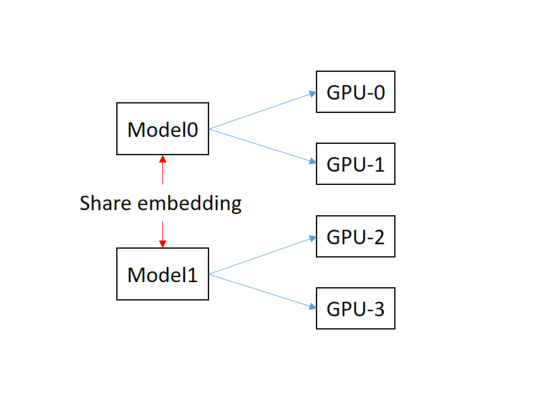# Distributed training for different models

The mdoel-0 is distributed trained in between GPU-0 and GPU-1 and model-1 works the same way on GPU-2 and GPU-3. In addition, the both models share word embedding parameters, which will be synchronously updated by them.

``````import torch

args.distributed_word_size=2
mp = torch.multiprocessing.get_context('spawn')
args.distributed_init_method_run_0 = 'tcp://localhost:00000'
args.distributed_init_method_run_1 = 'tcp://localhost:11111'

procs = []
for i in range(args.distributed_word_size):
args.distributed_rank = i
args.device_id = i
procs.append(mp.Process(target=run_0, args=(args, ), daemon=True))
procs[i].start()

for i in range(args.distributed_word_size):
args.distributed_rank = i
args.device_id = i + args.distributed_word_size
procs.append(mp.Process(target=run_1, args=(args, ), daemon=True))
procs[i+args.distributed_word_size].start()

for p in procs:
p.joint()

def run_0(args):
torch.distributed.init_process_group(
backend=args.distributed_backend,
init_method=args.distributed_init_method_run_0,
world_size=args.distributed_world_size,
rank=args.distributed_rank)
main_0(args)
....

def run_1(args):
torch.distributed.init_process_group(
backend=args.distributed_backend,
init_method=args.distributed_init_method_run_1,
world_size=args.distributed_world_size,
rank=args.distributed_rank)
main_1(args)
....

``````I have written a draft above. This draft take advantage of multiprocessing and distributed training for multi-GPUs in a single machine. The function of run_0 and run_1 refer to model-0 and model-1 respectively.

I wonder if the snippet above is correct and there is any better suggestion.
In particularly, how to implement the share word embedding for synchronously updating in two different models ?

Is there a specialist on pytorch ?